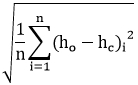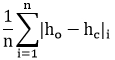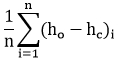# Fall Semester 2018

 Page 1 [Page 2] Page 3 Page 4 Page 5 Page 6

Multiple Choice

(1 pt each)

2. (calibration) Which of the following error norms tells you whether your computed heads are too high or too low on average?

 a. mean error (ME) b. mean absolute error (MAE) c. root mean squared error (RMS) d. none of the above.

3. (gms) When assigning a conductance value to an arc in the Map module of GMS as part of a MODFLOW conceptual model, the conductance should be entered as:

 a. Conductance^2 b. Conductance per cell c. Conductance per unit length d. Conductance per unit area

4. (calibration) Model non-uniqueness occurs when:

 a. One set of model input parameters will calibrate to multiple sets of observed data b. Multiple sets of model input parameters will calibrate to a single set of observed data c. Multiple sets of observed head and flow data are available, each of which were measured at different points in time d. The model input parameters will not calibrate to the available observed data

5. (calibration) Which of the following error norms is used by PEST as the objective function?

 a. Mean error b. Mean absolute error c. Root mean squared error d. Sum of the squared weighted residuals e. None of the above.

6. (calibration) Which of the following equations corresponds to the MAE error norm?

 a.b.c.d. None of the above.